• 微分变换构造雅可比逆解 注意：雅可比矩阵的构造和逆解的解算，此处只是理论，还需联系实际学习实验。 机器人微分运动原理： 1)机器人运动链中某一连杆对于固定系的位姿为T（T当前），经过微运动后该杆对固定系的...
微分变换构造雅可比逆解 注意：雅可比矩阵的构造和逆解的解算，此处只是理论，还需联系实际学习实验。 机器人微分运动原理： 1)机器人运动链中某一连杆对于固定系的位姿为T（T当前），经过微运动后该杆对固定系的位姿变为T+dT（T目标），若这个微运动是相对于固定系进行的，总可以用微小的平移和旋转来表示，即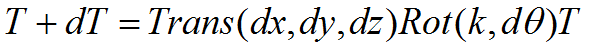T目*T-1=Trans(dx,dy,dz)*Rot(k,dθ)*T*T-1
Trans(dx,dy,dz)*Rot(k,dθ)=T目*T-1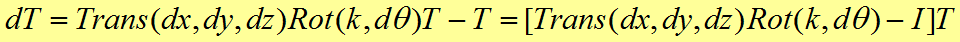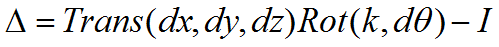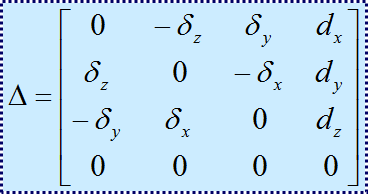注意：矩阵中各个不同符号代表是相对固定坐标系的的微分运动值（角度或位移量）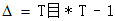2)若微分运动是相对于某个杆系{i}进行的，则T+dT可以表示为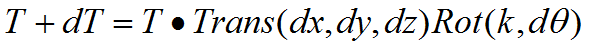T-1*T目=T-1*T*Trans(dx,dy,dz)*Rot(k,dθ)
Trans(dx,dy,dz)*Rot(k,dθ)=T-1*T目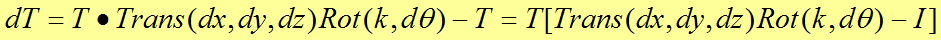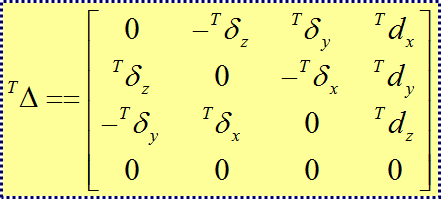注意：矩阵中左上角的T代表是相对坐标系T的微分运动值（角度或位移量）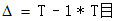1.目标矩阵：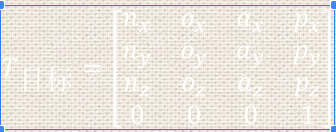2.根据当前各个关节角度，计算当前位姿矩阵T
3.由当前位姿和目标位姿计算微分运动矩阵：对于任一给定的坐标系T的微分运动矩阵：（矩阵中左上角的T代表是相对坐标系T的微分运动值）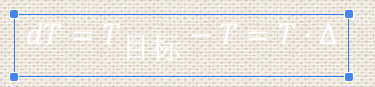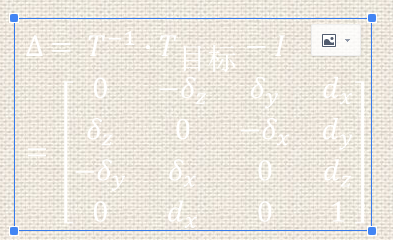4.根据微分运动矩阵，可以得到关节的微分运动D：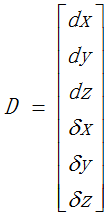5.构建雅可比矩阵： a.计算各连杆变换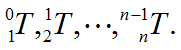b.计算各连杆至末端连杆的变换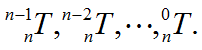c.计算J(q)的各列元素，第i列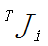由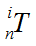所决定。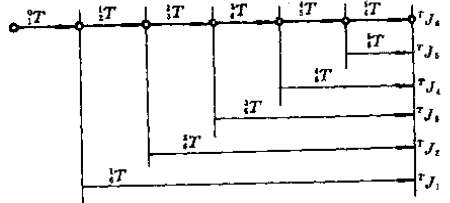若该关节为移动关节：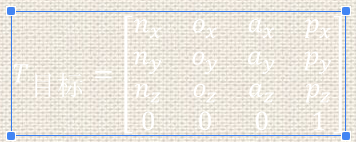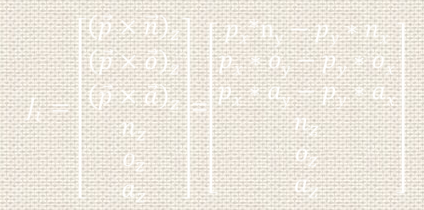6.根据雅可比矩阵，将一个坐标系内位置和姿态的微小变化变换为机器人手坐标系的位置和姿态的微小变化。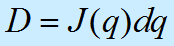即：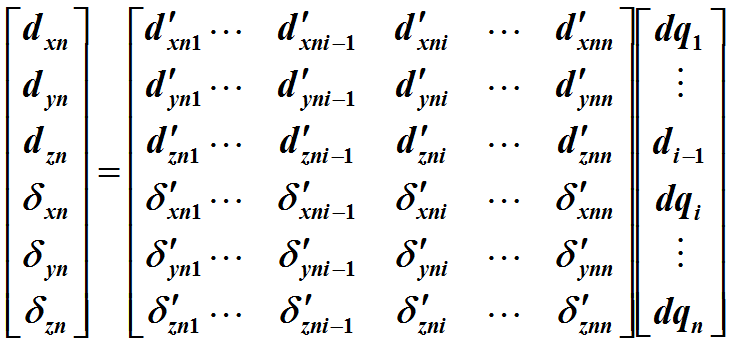7.关节变量=J(q)-1*D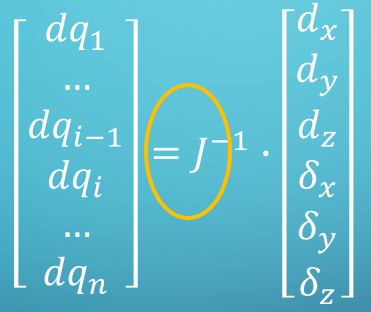注意：雅克比矩阵非满秩则无逆矩阵，故用svd分解求伪逆代替 8.设置阈值η和迭代次数N，进行迭代 1）当关节变量每个值的绝对值都小于η（η是满足精度需要的自定义小数）时，迭代结束，并输出当前各个关节值。 2）当迭代次数>N（N为满足预计迭代次数要求的自定义充分大数）时，迭代结束，并输出迭代失败信息 3）当关节变量>η且迭代次数小于N时，令θcur=θcur+dθ，并计算Tcur=Tθcur 9.重复2到8步骤，直至迭代退出条件满足
展开全文• 微分变换——机械臂的基座坐标系或者是末端执行器的坐标系发生微小的旋转和平移运动导致的末端执行器的位姿发生微小变换。 1.1基座坐标系下的变换 1.2末端执行器坐标系的变换 1.3微分运动的等价变换 1.1基座坐标系下...
微分变换——机械臂的基座坐标系或者是末端执行器的坐标系发生微小的旋转和平移运动导致的末端执行器的位姿发生微小变换。 1.1基座坐标系下的变换 1.2末端执行器坐标系的变换 1.3微分运动的等价变换
1.1基座坐标系下的变换 基座坐标系下的微分变换，是指相对于基座坐标系的微小平移或者旋转运动从而导致机械臂末端执行器的位姿发生变化。
T+dt=trans(dx,dy,dz)Rot(f,dθ)T
末端位姿为T  trans(dx,dy,dz)表示微分平移，Rot(f,dθ）表示微分旋转，由于是
参照的是基座坐标系，**所以是左乘微分变换矩阵。**
dt=(trans(dx,dy,dz)Rot(f,dθ)-1)T=Δ*T

在这里直接写出变换矩阵Δ
 Δ=[0   -δz  δy   dx;
δz   0   -δx  dy;
-δy  δx   0    dz;
0   0     0   1;]

1.2末端坐标系的微分变换 同理，是指相对于末端坐标系的微小运动导致的机械臂末端位姿发生变化。
T+dt=T trans(dx,dy,dz)Rot(f,dθ)
由于是相对末端执行器而言(自身),所以是使用矩阵右乘的方法，去求出之间的变换关系。
（T*TΔ）=dt=T(trans(dx,dy,dz)Rot(f,dθ)-1)
这里同样也有变换矩阵(TΔ)
(TΔ)=[0    -Tδz    Tδy   Tdx;
Tδz    0    -Tδx   Tdy;
-Tδy    Tδx    0    Tdz;
0     0     0      1;]

1.3微分运动的等价变换 微分变换的等价变换，是指基座坐标系的微分变换到末端执行器的微分变换之间的关系，一篇论文中说到，就是对于机器人末端的相对位姿变化，是从基座坐标系到末端坐标系的微分变换。
公式是（TΔ)=（T^-1）*（Δ）*(T) ，成立的条件是dt=Δ*T,dt=T*(TΔ);当他们等价时，就会成立。

先计算（Δ）(T)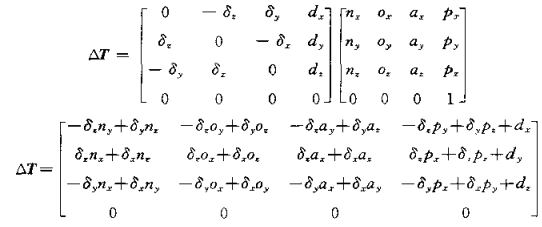由于（δn)z=-δny+δynz,以此类推，可以得到下面的等式（与上式等价）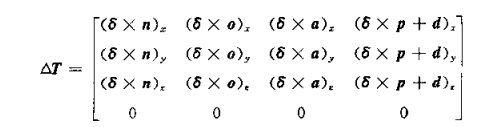然后是计算（T^-1)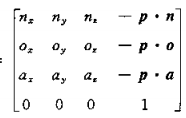接着根据上面的式子，我们可以的得到：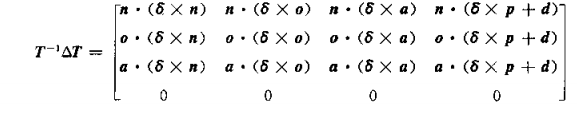同样依据等式(左=右）
(TΔ)=[0    -Tδz    Tδy   Tdx;
Tδz    0    -Tδx   Tdy;
-Tδy    Tδx    0    Tdz;
0     0     0      1;]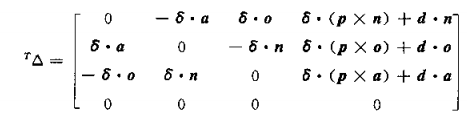这样子的话，我们就能得到：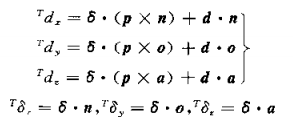根据上面的式子，我们还可以建立一个矩阵的等式：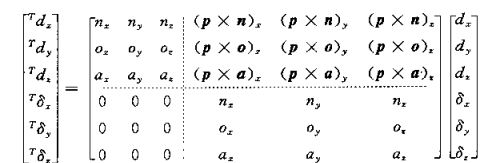具体是如何使用的，我们下回再讨论。
展开全文• 本文提出用无透镜傅里叶变换全息进行二阶光学微分的新方法,文中给出理论分析及实验结果。
• 但是我们这里也不准备解释什么是常微分方程了，直接进入本文实验的终极目标： 告诉你(y+x)dy+(x−y)dx=0(y+x)dy+(x-y)dx=0 然后问这个微分方程组的解是什么？ 有没有一脸懵13的感觉ahhh，这tm哪里是变量分离方程...
实验目标：
大家都是初学者嘛，既然会点进这种标题进来的话。但是我们这里也不准备解释什么是常微分方程了，直接进入本文实验的终极目标：

告诉你

(y+x)dy+(x−y)dx=0

(y+x)dy+(x-y)dx=0
然后问这个微分方程组的解是什么？

有没有一脸懵13的感觉ahhh，这tm哪里是变量分离方程了？emmm它确实不是，只不过我们这里也不准备花太多时间在变量分离方程上面（哎，要想解好变量分离方程建议大家去查查数学分析里面的求积分的方法更靠谱，这篇文章更多是在讲如何用变量变换把较一般的方程变成变量分离方程。）
先解释一下变量分离方程吧
emmm大家都知道万丈高楼平地起咯，虽然我觉得没有必要解释但是还是照顾一下初学者吧。变量分离方程大概就是长这个样子的：

dydx=f(x)φ(y)

\frac{dy}{dx}=f(x)\varphi(y)

有什么特点呢？特点就是这个方程特别容易解呀~很容易就可以将这个方程化为：

1φ(y)dy=f(x)dx

\frac{1}{\varphi(y)}dy=f(x)dx
这样的形式，然后我们再两边同时积分：

∫1φ(y)dy=∫f(x)dx

\int \frac{1}{\varphi(y)}dy=\int f(x)dx

接下来就是在考察大家的微积分知识了。
ok我们接下来进入正题，说一说一些常见的需要我们用些小手段进行才能进行变量分离的微分方程。
形如

dydx=g(yx)
$\frac{dy}{dx}=g(\frac{y}{x})$的形式转化为微分方程
其实这种方程叫齐次微分方程，我们用什么手段对其进行变换呢？让我思考一下。。。

先想好一个变换函数（这名字我自己想的），比如说

u=yx

u=\frac{y}{x}
然后我们在把这个变换函数给变换一个形式：

y=ux

y=ux
然后我们再对两边同时求导

dydx=u′⋅x+u

\frac{dy}{dx}=u'·x+u
看！我们又得到了一个

dydx
$\frac{dy}{dx}$的表达式，我们现在把它代入我们的标题中，就有：

u′⋅x+u=g(u)

u'·x+u=g(u)
这里已经得到一个变量分离函数啦~不造大家有米有看出来呀？没有看出来的话，我再化简一点点就有：

dudx=g(u)−ux

\frac{du}{dx}=\frac{g(u)-u}{x}
这不就是了吗？大家应该还记得怎么解吧···不记得的话自己看上面吧。

好的，有了这个变换—求导—重新代入的这几步小手段，我们可以轻而易举的搞定

dydx=g(yx)
$\frac{dy}{dx}=g(\frac{y}{x})$这种形式的微分方程，现在我们可以解决啥问题呢，比如

dydx=yx+tanyx
$\frac{dy}{dx}=\frac{y}{x}+tan\frac{y}{x}$，比如

xdydx+2xy−−√=y(x<0)
$x\frac{dy}{dx}+2\sqrt{xy}=y\quad (x<0)$  用以上所述的方法，这些基本上都只要做一些化简，或者根本就不用化简就可以搞定的微分方程。大家应该没有这需求，我也懒得在这里演示了。
接下来我们看看另外一种更为一般的且可以变量分离的微分方程：

dydx=a1x+b1y+c1a2x+b2y+c2

\frac{dy}{dx}=\frac{a_1x+b_1y+c_1}{a_2x+b_2y+c_2}
这样的形式。
显然我们之前所说的只不过是这个方程的一个特例而已。
那么这个样子的微分方程还可以用我们之前的套路吗？老实说不太行，我们来具体分析一下吧。
第一种情况：

a1a2=b1b2=c1c2=k
$\frac{a_1}{a_2}=\frac{b_1}{b_2}=\frac{c_1}{c_2}=k$的话那么有

dydx=k

\frac{dy}{dx}=k
接下来怎么做应该不用我说了吧
第二种情况：

a1a2=b1b2=k≠c1c2

\frac{a_1}{a_2}=\frac{b_1}{b_2}=k\neq \frac{c_1}{c_2}

这个时候怎么办呢？
构造变量分离方程

u=a2x+b2y
$u=a_2x+b_2y$
求导数有

dudx=a2+b2dydx

\frac{du}{dx}=a_2+b_2\frac{dy}{dx}
将原式代入进来：

dudx=a2+b2ku+c1u+c2

\frac{du}{dx}=a_2+b_2\frac{ku+c1}{u+c2}
这个东西是啥？它的右边只有

u
$u$而没有x$x$了啊，它就是一个变量分离方程。
好的，接下来看最后一种情况，这也是我们无法用以前的
变换—求导—重代入套路实现的一种情况：

a1a2≠b1b2

\frac{a_1}{a_2}\neq \frac{b_1}{b_2}
那我们怎么做呢，我们要想办法消去常数项，用给

x和y分别加上一个常数的方法
$x和y分别加上一个常数的方法$，为什么要消去常数项呢？我们来看看消去之后的效果，方程就可以变成：

dYdX=a1X+b1Ya2X+b2Y

\frac{dY}{dX}=\frac{a_1X+b_1Y}{a_2X+b_2Y}
这个是啥呀，这个就是

g(YX)
$g(\frac{Y}{X})$的形式呀，分子分母同时除以X就可以了。
那么现在问题来了，我们怎么样才能得到这个形式呢？不妨假设

X=x−α,Y=y−β
$X=x-\alpha,Y=y-\beta$，则原式可以变成：

dYdX=a1x+b1y−a1α−b1β+c1a2x+b2y−a2α−b2β+c2

\frac{dY}{dX}=\frac{a_1x+b_1y-a_1\alpha-b_1\beta+c_1}{a_2x+b_2y-a_2\alpha-b_2\beta+c_2}
然后求解出使得这个式子的常数项0的

α和β
$\alpha和\beta$就可以了。
求解出来之后就按照我们刚才所讲的思路用

dydx=g(yx)
$\frac{dy}{dx}=g(\frac{y}{x})$的解题方法进行求解就可以了。


展开全文• ## 微分方程

千次阅读 多人点赞 2017-10-11 09:37:21
微分方程的基本概念 可分离变量的微分方程 齐次方程 一阶线性微分方程 线性方程 可降阶的高阶微分方程 ynfxynfx型的微分方程 yfxyyfxy型的微分方程 yfyyyfyy型的微分方程 高阶线性微分方程 二阶线性微分方程举例...


微分方程的基本概念可分离变量的微分方程齐次方程一阶线性微分方程
线性方程 可降阶的高阶微分方程

y(n)=f(x)

y

(

n

)

=

f

(

x

)

$y^{(n)}=f(x)$型的微分方程

y″=f(x,y′)

y

″

=

f

(

x

,

y

′

)

$y''=f(x,y')$型的微分方程

y″=f(y,y′)

y

″

=

f

(

y

,

y

′

)

$y''=f(y,y')$型的微分方程 高阶线性微分方程
二阶线性微分方程举例线性微分方程的解的结构

微分方程的基本概念
表示未知函数、未知函数的导数与自变量之间的关系的方程，叫做微分方程，有时也简称方程微分方程中所出现的未知函数的最高阶导数的阶数，叫做微分方程的阶，如

x3y‴+x2y″−4xy′=3x2

x

3

y

‴

+

x

2

y

″

−

4

x

y

′

=

3

x

2

$x^3y'''+x^2y''-4xy'=3x^2$是三阶微分方程，

y(4)−4y‴+10y″−12y′+5y=sin2x

y

(

4

)

−

4

y

‴

+

10

y

″

−

12

y

′

+

5

y

=

sin

⁡

2

x

$y^{(4)}-4y'''+10y''-12y'+5y=\sin 2x$是四阶微分方程一般的，

n

n

$n$阶微分方程的形式是F(x,y,y′...,y(n))=0$F\left(x,y,{y}^{\prime }...,{y}^{\left(n\right)}\right)=0$F(x,y,y'...,y^{(n)})=0，其中

y(n)

y

(

n

)

$y^{(n)}$必须出现，而

x,y,y′,...,y(n−1)

x

,

y

,

y

′

,

.

.

.

,

y

(

n

−

1

)

$x,y,y',...,y^{(n-1)}$等变量则可以不出现，例如

n

n

$n$阶微分方程y(n)+1=0${y}^{\left(n\right)}+1=0$y^{(n)}+1=0首先建立微分方程，然后找出满足微分方程的函数（解微分方程），把这函数代入微分方程能使该方程称为恒等式。这个函数就叫做该微分方程的解如果微分方程的解中含有任意常数，且任意常数的个数与微分方程的阶数相同，这样的解叫做微分方程的通解。如

y=x2+C

y

=

x

2

+

C

$y=x^2+C$是

dydx=2x

d

y

d

x

=

2

x

$\frac{dy}{dx}=2x$的通解确定了通解中的任意常数以后，就得到微分方程的特解。例如

y=x2+1

y

=

x

2

+

1

$y=x^2+1$是

dydx=2x

d

y

d

x

=

2

x

$\frac{dy}{dx}=2x$满足条件

x=1

x

=

1

$x=1$时

y=2

y

=

2

$y=2$的特解求微分方程

y′=f(x,y)

y

′

=

f

(

x

,

y

)

$y'=f(x,y)$满足初始条件

y|x=x0=y0

y

|

x

=

x

0

=

y

0

$y|_{x=x_0}=y_0$的特解这样一个问题，叫做一阶微分方程的初值问题，记作

{y′=f(x,y)y|x=x0=y0

{

y

′

=

f

(

x

,

y

)

y

|

x

=

x

0

=

y

0

\left\{ \begin{aligned} y'=f(x,y) \\ y|_{x=x_0}=y_0 \end{aligned} \right. 微分方程的解的图形是一条曲线，叫做微分方程的积分曲线。上述初值问题的几何意义，就是求微分方程的通过点

(x0,y0)

(

x

0

,

y

0

)

$(x_0,y_0)$的那条积分曲线。二阶微分方程的初值问题

{y″=f(x,y,y′)y|x=x0=y0,y′|x=x0=y′0

{

y

″

=

f

(

x

,

y

,

y

′

)

y

|

x

=

x

0

=

y

0

,

y

′

|

x

=

x

0

=

y

0

′

\left\{ \begin{aligned} y''=f(x,y,y') \\ y|_{x=x_0}=y_0,y'|_{x=x_0}=y_0'\end{aligned}\right.的几何意义，是求微分方程的通过点

(x0,y0)

(

x

0

,

y

0

)

$(x_0,y_0)$且在该点处的切线斜率为

y′0

y

0

′

$y_0'$的那条积分曲线
可分离变量的微分方程
一般的，如果一个一阶微分方程能写成

g(y)dy=f(x)dx

g

(

y

)

d

y

=

f

(

x

)

d

x

g(y)dy=f(x)dx的形式，就是说，能把微分方程写成一端只含

y

y

$y$的函数和dy$dy$$dy$，另一端只含

x

x

$x$的函数和dx$dx$$dx$，那么原方程就称为可分离变量的微分方程例：求微分方程

dydx=2xy(1)

(1)

d

y

d

x

=

2

x

y

\frac{dy}{dx}=2xy \tag{1}的通解：  上述方程式可分离变量的，分离变量后得：

dyy=2xdx

d

y

y

=

2

x

d

x

\frac{dy}{y}=2xdx，两端积分：

∫dyy=∫2xdx

∫

d

y

y

=

∫

2

x

d

x

$\int \frac{dy}{y}=\int 2xdx$，得

ln|y|=x2+C1

ln

⁡

|

y

|

=

x

2

+

C

1

$\ln|y|=x^2+C_1$，从而

y=±ex2+C1=±eC1ex2

y

=

±

e

x

2

+

C

1

=

±

e

C

1

e

x

2

$y=\pm e^{x^2+C_1}=\pm e^{C_1}e^{x^2}$。因为

±eC1

±

e

C

1

$\pm e^{C_1}$是任意非零常数，又

y≡0

y

≡

0

$y \equiv0$也是方程（1）的解；故得方程（7）的通解

y=Cex2

y

=

C

e

x

2

y=Ce^{x^2}
齐次方程
如果一阶微分方程可化为

dydx=φ(yx)(1)

(1)

d

y

d

x

=

φ

(

y

x

)

\frac{dy}{dx}=\varphi (\frac{y}{x}) \tag{1}的形式，那么就称这方程为齐次方程。例如

(xy−y2)dx−(x2−2xy)dy=0

(

x

y

−

y

2

)

d

x

−

(

x

2

−

2

x

y

)

d

y

=

0

(xy-y^2)dx-(x^2-2xy)dy=0是齐次方程，因为它可化成

dydx=yx−(yx)21−2(yx)

d

y

d

x

=

y

x

−

(

y

x

)

2

1

−

2

(

y

x

)

\frac{dy}{dx}=\frac{\frac{y}{x}-(\frac{y}{x})^2}{1-2(\frac{y}{x})}例：解方程

y2+x2dydx=xydydx

y

2

+

x

2

d

y

d

x

=

x

y

d

y

d

x

y^2+x^2\frac{dy}{dx}=xy\frac{dy}{dx}，原方程可写成

dydx=y2xy−x2=(yx)2yx−1

d

y

d

x

=

y

2

x

y

−

x

2

=

(

y

x

)

2

y

x

−

1

\frac{dy}{dx}=\frac{y^2}{xy-x^2}=\frac{(\frac{y}{x})^2}{\frac{y}{x}-1}，因此是齐次方程，令

yx=u

y

x

=

u

$\frac{y}{x}=u$，则

y=ux，dydx=u+xdudx

y

=

u

x

，

d

y

d

x

=

u

+

x

d

u

d

x

y=ux，\frac{dy}{dx}=u+x\frac{du}{dx}，于是原方程变为

xdudx=uu−1

x

d

u

d

x

=

u

u

−

1

x\frac{du}{dx}=\frac{u}{u-1}，分离变量得

u−ln|u|+C=ln|x|

u

−

ln

⁡

|

u

|

+

C

=

ln

⁡

|

x

|

u-\ln|u|+C=\ln|x|，或写为

ln|xu|=u+C

ln

⁡

|

x

u

|

=

u

+

C

\ln|xu|=u+C，将

yx

y

x

$\frac{y}{x}$代入上式中的

u

u

$u$，便得到所给方程的通解为ln|y|=yx+C$\mathrm{ln}|y|=\frac{y}{x}+C$\ln|y|=\frac{y}{x}+C
一阶线性微分方程
线性方程
方程

dydx+P(x)y=Q(x)(1)

(1)

d

y

d

x

+

P

(

x

)

y

=

Q

(

x

)

\frac{dy}{dx}+P(x)y=Q(x) \tag{1}叫做一阶线性微分方程，因为它对于未知函数

y

y

$y$及其导数是一次方程。如果Q(x)≡0$Q\left(x\right)\equiv 0$$Q(x)\equiv 0$，则方程（1）称为齐次的；如果

Q(x)≠0

Q

(

x

)

≠

0

$Q(x)\not=0$，则方程（1）称为非齐次的设（1）为非齐次线性方程，为了求出非齐次线性方程（1）的解，先把

Q(x)

Q

(

x

)

$Q(x)$换成零而写出方程

dydx+P(x)y=0(2)

(2)

d

y

d

x

+

P

(

x

)

y

=

0

\frac{dy}{dx}+P(x)y=0\tag{2}，方程（2）叫做对应于非齐次线性方程（1）的齐次线性方程。方程（2）是可分离变量的，分离变量后得

dyy=−P(x)dx

d

y

y

=

−

P

(

x

)

d

x

\frac{dy}{y}=-P(x)dx，两端积分，得

ln|y|=0∫P(x)dx+C1

ln

⁡

|

y

|

=

0

∫

P

(

x

)

d

x

+

C

1

\ln|y|=0\int P(x)dx+C_1，或

y=Ce−∫P(x)dx（C=±eC1）

y

=

C

e

−

∫

P

(

x

)

d

x

（

C

=

±

e

C

1

）

y=Ce^{-\int P(x)dx}（C=\pm e^{C_1}），这是对应的齐次线性方程（2）的通解使用常数变易法来求非齐次线性方程（1）的通解，将（2）的通解中的

C

C

$C$换成x$x$$x$的未知函数

u(x)

u

(

x

)

$u(x)$，即作变换

y=ue−∫P(x)dx(3)

(3)

y

=

u

e

−

∫

P

(

x

)

d

x

y=ue^{-\int P(x)dx} \tag{3}，于是

dydx=u′e−∫P(x)dx−uP(x)e−∫P(x)dx(4)

(4)

d

y

d

x

=

u

′

e

−

∫

P

(

x

)

d

x

−

u

P

(

x

)

e

−

∫

P

(

x

)

d

x

\frac{dy}{dx}=u'e^{-\int P(x)dx}-uP(x)e^{-\int P(x)}dx \tag{4}，将（3）和（4）代入方程（1）得

u′e−∫P(x)dx−uP(x)e−∫P(x)dx+P(x)ue−∫P(x)dx=Q(x)

u

′

e

−

∫

P

(

x

)

d

x

−

u

P

(

x

)

e

−

∫

P

(

x

)

d

x

+

P

(

x

)

u

e

−

∫

P

(

x

)

d

x

=

Q

(

x

)

u'e^{-\int P(x)dx}-uP(x)e^{-\int P(x)dx}+P(x)ue^{-\int P(x)dx}=Q(x)，即

u′e−∫P(x)dx=Q(x)，u′=Q(x)e∫P(x)dx

u

′

e

−

∫

P

(

x

)

d

x

=

Q

(

x

)

，

u

′

=

Q

(

x

)

e

∫

P

(

x

)

d

x

u'e^{-\int P(x)dx}=Q(x)，u'=Q(x)e^{\int P(x)dx}，两端积分，得

u=∫Q(x)e∫P(x)dxdx+C

u

=

∫

Q

(

x

)

e

∫

P

(

x

)

d

x

d

x

+

C

u=\int Q(x)e^{\int P(x)dx}dx+C，把上式代入（3），便得非齐次线性方程（1）的通解

y=e−∫P(x)dx(∫Q(x)e∫P(x)dxdx+C)(5)

(5)

y

=

e

−

∫

P

(

x

)

d

x

(

∫

Q

(

x

)

e

∫

P

(

x

)

d

x

d

x

+

C

)

y=e^{-\int P(x)dx}(\int Q(x)e^{\int P(x)dx}dx+C) \tag{5}，把（5）式改写成两项之和

y=Ce−∫P(x)dx+e−∫P(x)dx∫Q(x)e∫P(x)dxdx

y

=

C

e

−

∫

P

(

x

)

d

x

+

e

−

∫

P

(

x

)

d

x

∫

Q

(

x

)

e

∫

P

(

x

)

d

x

d

x

y=Ce^{-\int P(x)dx}+e^{-\int P(x)dx}\int Q(x)e^{\int P(x)dx}dx由此可知，一阶非齐次线性方程的通解等于对应的齐次方程的通解与非齐次方程的一个特解之和例：求方程

dydx−2yx+1=(x+1)52

d

y

d

x

−

2

y

x

+

1

=

(

x

+

1

)

5

2

\frac{dy}{dx}-\frac{2y}{x+1}=(x+1)^{\frac{5}{2}}的通解.  解：这是一个非齐次线性方程，先求对应的齐次方程的通解.

dydx−2x+1y=0，

d

y

d

x

−

2

x

+

1

y

=

0

，

\frac{dy}{dx}-\frac{2}{x+1}y=0，

dyy=2dxx+1，

d

y

y

=

2

d

x

x

+

1

，

\frac{dy}{y}=\frac{2dx}{x+1}，

ln|y|=2ln|x+1|+ln|C1|，

ln

⁡

|

y

|

=

2

ln

⁡

|

x

+

1

|

+

ln

⁡

|

C

1

|

，

\ln|y|=2\ln|x+1|+\ln|C_1|，

y=C(x+1)2(6)

(6)

y

=

C

(

x

+

1

)

2

y=C_(x+1)^2 \tag{6}，那么

dydx=u′(x+1)2+2u(x+1)

d

y

d

x

=

u

′

(

x

+

1

)

2

+

2

u

(

x

+

1

)

\frac{dy}{dx}=u'(x+1)^2+2u(x+1)，代入所给非齐次方程，得

u′=(x+1)12

u

′

=

(

x

+

1

)

1

2

u'=(x+1)^{\frac{1}{2}}，两端积分，得

u=23(x+1)32+C

u

=

2

3

(

x

+

1

)

3

2

+

C

u=\frac{2}{3}(x+1)^{\frac{3}{2}}+C，再把上式代入（6）式，即得所求方程的通解为

y=(x+1)2[23(x+1)32+C]

y

=

(

x

+

1

)

2

[

2

3

(

x

+

1

)

3

2

+

C

]

y=(x+1)^2[\frac{2}{3}(x+1)^\frac{3}{2}+C]
可降阶的高阶微分方程
二阶及二阶以上的微分方程，即所谓高阶微分方程

y(n)=f(x)

y

(

n

)

=

f

(

x

)

$y^{(n)}=f(x)$型的微分方程
微分方程

y(n)=f(x)(2)

(2)

y

(

n

)

=

f

(

x

)

y^{(n)}=f(x) \tag{2}的右端仅含有自变量

x

x

$x$，容易看出，只要把y(n−1)${y}^{\left(n-1\right)}$$y^{(n-1)}$作为新的未知函数，那么（2）式就是新未知函数的一阶微分方程。两边积分，就得到一个

n−

n

−

$n-$阶的微分方程

y(n−1)=∫f(x)dx+C1

y

(

n

−

1

)

=

∫

f

(

x

)

d

x

+

C

1

y^{(n-1)}=\int f(x)dx+C_1，同理可得

y(n−2)=∫[∫f(x)dx+C1]dx+C2

y

(

n

−

2

)

=

∫

[

∫

f

(

x

)

d

x

+

C

1

]

d

x

+

C

2

y^{(n-2)}=\int[\int f(x)dx+C_1]dx+C_2，依此法继续进行，接连积分

n

n

$n$次，便得到方程（2）的含有n$n$$n$个任意常数的通解例：求微分方程

y‴=e2x−cosx

y

‴

=

e

2

x

−

cos

⁡

x

y'''=e^{2x}-\cos x的通解  解：对所给方程接连积分三次，得：

y″=12e2x−sinx+C

y

″

=

1

2

e

2

x

−

sin

⁡

x

+

C

y''=\frac{1}{2}e^{2x}-\sin x+C，

y′=14e2x+cosx+Cx+C2

y

′

=

1

4

e

2

x

+

cos

⁡

x

+

C

x

+

C

2

y'=\frac{1}{4}e^{2x}+\cos x+Cx+C_2，

y=18e2x+sinx+C1x2+C2x+C3（C1=C2）

y

=

1

8

e

2

x

+

sin

⁡

x

+

C

1

x

2

+

C

2

x

+

C

3

（

C

1

=

C

2

）

y=\frac{1}{8}e^{2x}+\sin x+C_1x^2+C_2x+C_3（C_1=\frac{C}{2}）这就是所求的通解

y″=f(x,y′)

y

″

=

f

(

x

,

y

′

)

$y''=f(x,y')$型的微分方程
方程

y″=f(x,y′)(7)

(7)

y

″

=

f

(

x

,

y

′

)

y''=f(x,y') \tag{7}的右端不显含未知函数

y

y

$y$。如果我们设y′=p${y}^{\prime }=p$$y'=p$，那么

y″=dpdx=p′

y

″

=

d

p

d

x

=

p

′

y''=\frac{dp}{dx}=p'，而方程（7）就成为

p′=f(x,p)

p

′

=

f

(

x

,

p

)

p'=f(x,p)。这是一个关于变量

x、p

x

、

p

$x、p$的一阶微分方程，设其通解为

p=φ(x,C1)

p

=

φ

(

x

,

C

1

)

p=\varphi(x,C_1)，但是

p=dydx

p

=

d

y

d

x

$p=\frac{dy}{dx}$，因此又得到一个一阶微分方程

dydx=φ(x,C1)

d

y

d

x

=

φ

(

x

,

C

1

)

\frac{dy}{dx}=\varphi(x,C_1)，对它进行积分，便得到方程（7）的通解为

y=∫φ(x,C1)dx+C2

y

=

∫

φ

(

x

,

C

1

)

d

x

+

C

2

y=\int \varphi(x,C_1)dx+C_2例：求微分方程

(1+x2)y″=2xy′

(

1

+

x

2

)

y

″

=

2

x

y

′

(1+x^2)y''=2xy'满足初始条件

y|x=0=1，y′|x=0=3

y

|

x

=

0

=

1

，

y

′

|

x

=

0

=

3

y|_{x=0}=1，y'|_{x=0}=3的特解  解：所给方程式

y″=f(x,y′)

y

″

=

f

(

x

,

y

′

)

$y''=f(x,y')$型的，设

y′=p

y

′

=

p

$y'=p$，代入方程并分离变量后，有

dpp=2x1+x2dx

d

p

p

=

2

x

1

+

x

2

d

x

\frac{dp}{p}=\frac{2x}{1+x^2}dx两端积分，得

ln|p|=ln(1+x2)+C，

ln

⁡

|

p

|

=

ln

⁡

(

1

+

x

2

)

+

C

，

\ln|p|=\ln(1+x^2)+C，即

p=y′=C1(1+x2)（C1=±eC）

p

=

y

′

=

C

1

(

1

+

x

2

)

（

C

1

=

±

e

C

）

p=y'=C_1(1+x^2)（C_1=\pm e^C），由条件

y′|x=0=3

y

′

|

x

=

0

=

3

$y'|_{x=0}=3$，得

C1=3，

C

1

=

3

，

C_1=3，所以

y′=3(1+x2)，

y

′

=

3

(

1

+

x

2

)

，

y'=3(1+x^2)，两端再积分，得

y=x3+3x+C2

y

=

x

3

+

3

x

+

C

2

y=x^3+3x+C_2。又由条件

y|x=0=1

y

|

x

=

0

=

1

$y|_{x=0}=1$，得

C2=1

C

2

=

1

C_2=1，于是所求的特解为

y=x3+3x+1

y

=

x

3

+

3

x

+

1

y=x^3+3x+1

y″=f(y,y′)

y

″

=

f

(

y

,

y

′

)

$y''=f(y,y')$型的微分方程
方程

y″=f(y,y′)(11)

(11)

y

″

=

f

(

y

,

y

′

)

y''=f(y,y') \tag{11}中不明显地含自变量

x

x

$x$，为了求出它的解，我们令y′=p${y}^{\prime }=p$$y'=p$，并利用复合函数的求导法则把

y″

y

″

$y''$化为对

y

y

$y$的导数，即y″=dpdx=dpdy⋅dydx=pdpdy${y}^{″}=\frac{dp}{dx}=\frac{dp}{dy}\cdot \frac{dy}{dx}=p\frac{dp}{dy}$y''=\frac{dp}{dx}=\frac{dp}{dy}\cdot \frac{dy}{dx}=p\frac{dp}{dy}，这样，方程（11）就成为

pdpdy=f(y,p)

p

d

p

d

y

=

f

(

y

,

p

)

p\frac{dp}{dy}=f(y,p)这是一个关于变量

y、p

y

、

p

$y、p$的一阶微分方程，设它的通解为

y′=p=φ(y,C1)

y

′

=

p

=

φ

(

y

,

C

1

)

y'=p=\varphi(y,C_1)，分离变量并积分，便得方程（11）的通解为

∫dyφ(y,C1)=x+C2

∫

d

y

φ

(

y

,

C

1

)

=

x

+

C

2

\int\frac{dy}{\varphi(y,C_1)}=x+C_2例：求微分方程

yy″−y′2=0(12)

(12)

y

y

″

−

y

′

2

=

0

yy''-y'^2=0 \tag{12}的通解  解：方程（12）不明显地含自变量

x

x

$x$，设y′=p，则y″=pdpdy，${y}^{\prime }=p，则{y}^{″}=p\frac{dp}{dy}，$y'=p，则y''=p\frac{dp}{dy}，代入方程（12），得

ypdpdy−p2=0

y

p

d

p

d

y

−

p

2

=

0

yp\frac{dp}{dy}-p^2=0，在

y≠0、p≠0

y

≠

0

、

p

≠

0

$y\not=0、p\not=0$时，约去

p

p

$p$并分离变量，得dpp=dyy$\frac{dp}{p}=\frac{dy}{y}$\frac{dp}{p}=\frac{dy}{y}。两端积分，得

ln|p|=ln|y|+C

ln

⁡

|

p

|

=

ln

⁡

|

y

|

+

C

\ln|p|=\ln|y|+C，即

p=C1y，或y′=C1y（C1=±eC）

p

=

C

1

y

，

或

y

′

=

C

1

y

（

C

1

=

±

e

C

）

p=C_1y，或y'=C_1y（C_1=\pm e^C）再分离变量并两端积分，便得方程（12）的通解为

ln|y|=C1x+C′2

ln

⁡

|

y

|

=

C

1

x

+

C

2

′

\ln|y|=C_1x+C_2'，或

y=C2eC1x（C2=±eC′2）

y

=

C

2

e

C

1

x

（

C

2

=

±

e

C

2

′

）

y=C_2e^{C_1x}（C_2=\pm e^{C_2'}）
高阶线性微分方程
二阶线性微分方程举例
如方程

d2ydx2+P(x)dydx+Q(x)y=f(x)

d

2

y

d

x

2

+

P

(

x

)

d

y

d

x

+

Q

(

x

)

y

=

f

(

x

)

\frac{d^2y}{dx^2}+P(x)\frac{dy}{dx}+Q(x)y=f(x)叫做二阶线性微分方程，当方程右端

f(x)≡0

f

(

x

)

≡

0

$f(x)\equiv0$时，方程叫做齐次的；当

f(x)≠0

f

(

x

)

≠

0

$f(x)\not= 0$时，方程叫做非齐次的

线性微分方程的解的结构
对于二阶齐次线性方程

y″+P(x)y′+Q(x)y=0(6)

(6)

y

″

+

P

(

x

)

y

′

+

Q

(

x

)

y

=

0

y''+P(x)y'+Q(x)y=0 \tag{6}
1. 如果函数

y′1(x)

y

1

′

(

x

)

$y_1'(x)$与

y2(x)

y

2

(

x

)

$y_2(x)$是方程（6）的两个解，那么

y=C1y1(x)+C2y2(x)(7)

(7)

y

=

C

1

y

1

(

x

)

+

C

2

y

2

(

x

)

y=C_1y_1(x)+C_2y_2(x) \tag{7}也是（6）的解，其中

C1、C2

C

1

、

C

2

$C_1、C_2$是任意常数
2. 设

y1(x),y2(x),...,yn(x)

y

1

(

x

)

,

y

2

(

x

)

,

.

.

.

,

y

n

(

x

)

$y_1(x),y_2(x),...,y_n(x)$为定义在区间

I

I

$I$上的n$n$$n$个函数，如果存在

n

n

$n$个不全为零的常数k1,k2,...,kn${k}_{1},{k}_{2},...,{k}_{n}$$k_1,k_2,...,k_n$，使得当

x∈I

x

∈

I

$x\in I$时有恒等式

k1y1+k2y2+...+knyn≡0

k

1

y

1

+

k

2

y

2

+

.

.

.

+

k

n

y

n

≡

0

k_1y_1+k_2y_2+...+k_ny_n\equiv 0成立，那么称这

n

n

$n$个函数在区间I$I$$I$上线性相关；否则称线性无关
3. 如果

y1(x)

y

1

(

x

)

$y_1(x)$与

y2(x)

y

2

(

x

)

$y_2(x)$是方程（6）的两个线性无关的特解，那么

y=C1y1(x)+C2y2(x)（C1、C2是任意常数）

y

=

C

1

y

1

(

x

)

+

C

2

y

2

(

x

)

（

C

1

、

C

2

是

任

意

常

数

）

y=C_1y_1(x)+C_2y_2(x)（C_1、C_2是任意常数）就是方程（6）的通解
4. 如果

y1(x),y2(x),...,yn(x)

y

1

(

x

)

,

y

2

(

x

)

,

.

.

.

,

y

n

(

x

)

$y_1(x),y_2(x),...,y_n(x)$是

n

n

$n$阶齐次线性方程y(n)+a1(x)y(n−1)+...+an−1(x)y′+an(x)y=0${y}^{\left(n\right)}+{a}_{1}\left(x\right){y}^{\left(n-1\right)}+...+{a}_{n-1}\left(x\right){y}^{\prime }+{a}_{n}\left(x\right)y=0$y^{(n)}+a_1(x)y^{(n-1)}+...+a_{n-1}(x)y'+a_n(x)y=0的

n

n

$n$个线性无关的解，那么，此方程的通解为y=C1y1(x)+C2y2(x)+...+Cnyn(x)$y={C}_{1}{y}_{1}\left(x\right)+{C}_{2}{y}_{2}\left(x\right)+...+{C}_{n}{y}_{n}\left(x\right)$y=C_1y_1(x)+C_2y_2(x)+...+C_ny_n(x)，其中

C1,C2,...,Cn

C

1

,

C

2

,

.

.

.

,

C

n

$C_1,C_2,...,C_n$为任意常数
5. 设

y∗(x)

y

∗

(

x

)

$y^*(x)$是二阶非齐次线性方程

y″+P(x)y′+Q(x)y=f(x)(5)

(5)

y

″

+

P

(

x

)

y

′

+

Q

(

x

)

y

=

f

(

x

)

y''+P(x)y'+Q(x)y=f(x) \tag{5}的一个特解，

Y(x)

Y

(

x

)

$Y(x)$是与（5）对应的齐次方程（6）的通解，那么

y=Y(x)+y∗(x)(8)

(8)

y

=

Y

(

x

)

+

y

∗

(

x

)

y=Y(x)+y^*(x)\tag{8}是二阶非齐次线性微分方程（5）的通解
6. 设非齐次线性方程（5）的右端

f(x)

f

(

x

)

$f(x)$是两个函数之和，即

y″+P(x)y′+Q(x)y=f1(x)+f2(x)，(9)

(9)

y

″

+

P

(

x

)

y

′

+

Q

(

x

)

y

=

f

1

(

x

)

+

f

2

(

x

)

，

y''+P(x)y'+Q(x)y=f_1(x)+f_2(x)\tag{9}，而

y∗1(x)

y

1

∗

(

x

)

$y_1^*(x)$与

y2∗(x)

y

2

∗

(

x

)

$y_2*(x)$分别是方程

y″+P(x)y′+Q(x)y=f1(x)

y

″

+

P

(

x

)

y

′

+

Q

(

x

)

y

=

f

1

(

x

)

y''+P(x)y'+Q(x)y=f_1(x)与

y″+P(x)y′+Q(x)y=f2(x)

y

″

+

P

(

x

)

y

′

+

Q

(

x

)

y

=

f

2

(

x

)

y''+P(x)y'+Q(x)y=f_2(x)的特解，那么

y∗1(x)+y∗2(x)

y

1

∗

(

x

)

+

y

2

∗

(

x

)

$y_1^*(x)+y_2^*(x)$就是原方程的特解

展开全文数学
• 数值计算微分，即取极限求导数 符号微分，使用表达式来计算导数 自动微分 比如下面的程序所示 import numpy as np def sigmoid(x): """ Compute sigmoid Function """ return 1 / (1+np.exp(-x)) def ...
• 文章目录写在前面坐标系的微分运动微分平移绕参考轴xyz的微分旋转绕一般轴q的微分旋转坐标系的微分变换微分运动算子：相对于固定参考坐标系、相对于自身坐标系 写在前面 微分运动指机构（例如机器人）的微小运动，...机器人学 雅可比矩阵
• 文章目录#一阶经典法##可分离变量型##不可分\齐次##一阶线性微分方程#二阶经典法##可降阶二阶##二阶常系数齐次线性微分方程##二阶常系数非齐次线性微分方程#微分算子法 D 求特解#积分变换微分方程 欢迎纠错 #一阶...高等数学 复变函数
• ## 常微分方程 笔记

千次阅读 多人点赞 2019-06-04 19:37:25
微分方程 笔记 ...一般形式：F(x,y,dydx,…,dnydxn)=0F(x,y,\frac{dy}{dx},\dots,\frac{d^ny}{dx^n})=0F(x,y,dxdy​,…,dxndny​)=0 线性与非线性 定义：若方程 F(x,y,dydx,…,dnydxn)=0F(x,y,\fr...
• 微分方程：描述自然界中存在的物理现象和普遍规律。 常微分方程（ODE） 偏微分方程（PDE） 偏微分方程理论： 物理/工程问题————翻译（建模）/物理工程规律————》数学问题（PDE） 物理/工程问题————...
• 傅里叶变换使任一信号可以有两种描述形式：时域描述和频域描述。 1. 线性 x1(t)↔FX1(w)x2(t)↔FX2(w) x_1(t)\overset{F}{\leftrightarrow}X_1(w) \\ x_2(t)\overset{F}{\leftrightarrow}X_2(w) x1​(t)↔FX1​(w)x2...
• ## 拟微分算子

千次阅读 2017-03-29 21:16:11
微分算子的研究开始于1960年代，最开始见于 Kohn，Hormander 等人的工作。拟微分算子在Atiyah-Singer 指标定理证明扮演...常系数的线性微分算子：P(D):=∑αaαDα(1)P(D):=\sum_{\alpha}a_{\alpha}D^{\alpha}\tag{1}
• 线性微分方程，是指以下形式的微分方程：   其中微分算子L是线性算子，y是一个未知的函数，等式的右面...其中D微分算子d/dx（也就是Dy = y'，Dy = y"，……），ai是给定的函数。这个微分方程是n阶的，因为方程
• 写在前面微分运动指机构（例如机器人）的微小运动，可以用它来推导不同部件之间的速度关系。依据定义，微分运动就是微小的运动。因此，如果能够在一个很小的时间段内测量或者计算这个运动，就能得到速度关系。为啥想...
• 浅谈傅里叶变换、小波变换、HHT变换一、傅里叶变换1.1傅里叶变换介绍二、小波变换2.1小波变换正反变换公式2.2小波变换适应场景及其优缺点2.3小波变换的应用三、HHT变换3.1HHT产生的背景3.1 HHT变换介绍3.2 HHT对信号...
• #从RN\mathbb{R}^{N}RN到 RM\mathbb{R}^{M}RM映射的微分(向量函数的微分) 国内的数学分析教材(就我所知)基本上不讲向量函数的微分，就更不用指望高等代数里有了，但是国外的数学分析或者微积分里却常常会提到这一...向量函数
• 使用拉普拉斯变换解一阶、二阶微分方程。 一、核心内容 拉氏变换对 时域 s域 eat\displaystyle e^{at}eat 1s−a\displaystyle \frac{1}{s-a}s−a1​ 1\displaystyle 11 1s\displaystyle \frac{1}{s}s1​...
• ## 微分几何I

千次阅读 2018-08-01 00:18:08
• 我们可以从多次积分 、积分变换、广义函数、常微分方程 ,以及类似经典积分微分作为“和”与“差”的 极限等各种途径来定义 R-L 分数积分与微分 .设 ν∈(0 , 1), a , b∈R, a , f (t)在[ a , b] 上连续 ,定 义 R-L ...
• 脉冲函数 (d (t) - delta of dirac)、阶跃函数 u (t) 和斜坡函数 t * u (t) 通过使用傅立叶变换。 图形和符号形式的差异方程显示三种类型的响应，脉冲，阶跃和斜坡，输入方程的系数和响应的系数，使用stem以图形...matlab
• ## 深入理解傅里叶变换

万次阅读 多人点赞 2018-05-31 13:03:37
这原本是我在知乎上对傅立叶变换、拉普拉斯变换、Z变换的联系？为什么要进行这些变换。研究的都是什么？问题的回答，实际上是我在本科学习数学和信号处理期间的思考... 此外部分地方要求读者有一定的微分方程基础，...
• §5 微分方程稳定性 C1 稳定性 1）平衡解/驻定解：令驻定微分方程右端为0得到的常数解 2）稳定性： 稳定：∀ϵ>0,∃δ(ϵ,t0)>0,s.t.∥x(t0)∥≤δ  ⟹  ∀t≥t0,∥x(t)∥<ϵ\forall \epsilon\gt0,\...
• 注：字母D表示求微分，D2、D3等表示求高阶微分D后所跟的字母为因变量，自变量可以指定或由系统规则选定为缺省。 如，微分方程d2y/dx2 =0 应表达为：D2y=0 例1：求 du/dt=1+u2的通解。 输入命令：dsolve(‘Du=1+u^2...
• ## Park变换及Park逆变换

万次阅读 多人点赞 2019-05-08 10:11:25
由于PID控制器对直流参考信号的跟踪效果更好，因此在Clark变换之后需要将静止的α,β坐标系转换为旋转的d,q坐标系（Park变换也称2s/2r变换）。 SVPWM算法的实现用的是静止的坐标系α,β，因此得到id,iq进行完PID...
• 我们都知道欧拉方程是比较简单的变系数微分方程，但如果产生了D算符的延迟，则又该将如何求解？让我来告诉你吧！相信我没错！！...